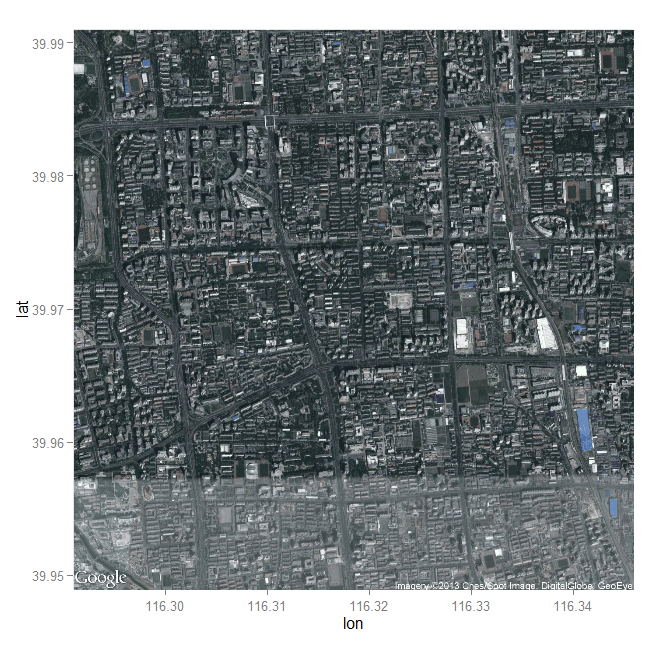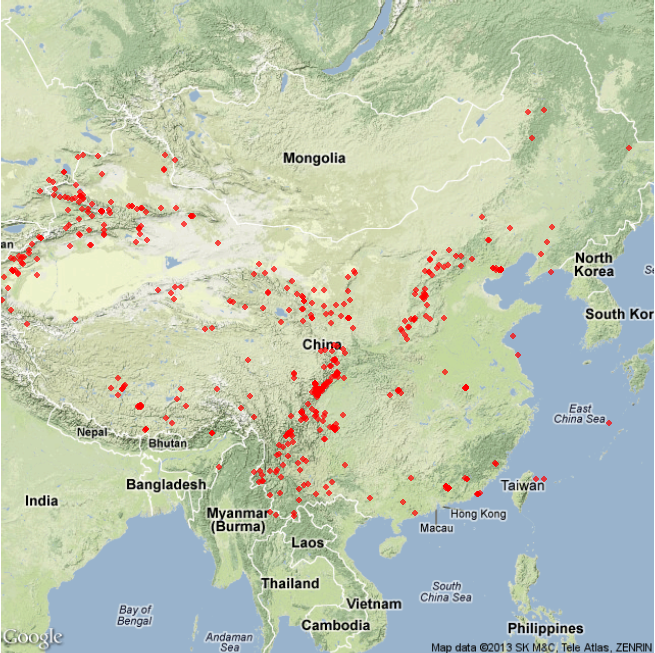1. 你得需要绘制地图；（约等于废话）
2. 你得有要绘制地图的地理信息，经纬度啊，边界啊等等；
3. 你得利用2的数据在R中画出来。

R语言中绘制地图的思路也是由于2的获取方式不一样而分开的。

## 1、 画世界地图

``````  install.packages('maps')
``````

``````library(maps)
map("world", fill = TRUE, col = rainbow(200),
ylim = c(-60, 90), mar = c(0, 0, 0, 0))
title("世界地图")
``````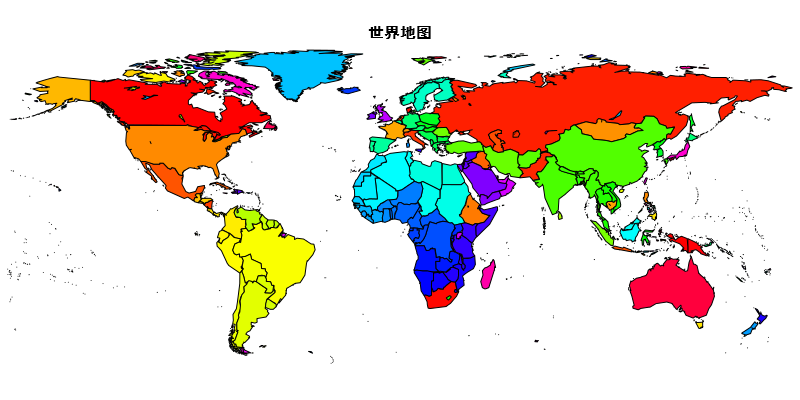## 2、 画美国地图

``````library(maps)
map("state", fill = TRUE, col = rainbow(209),
mar = c(0, 0, 2, 0))
title("美国地图")
``````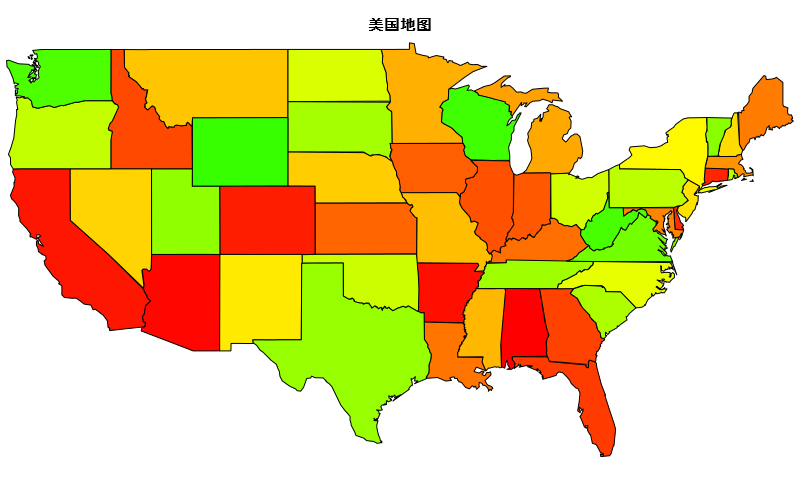``````library(maps)
map('state', region = c('new york', 'new jersey', 'penn'),
fill = TRUE, col = rainbow(3), mar = c(2, 3, 4, 3))
title("美国三州地图")
``````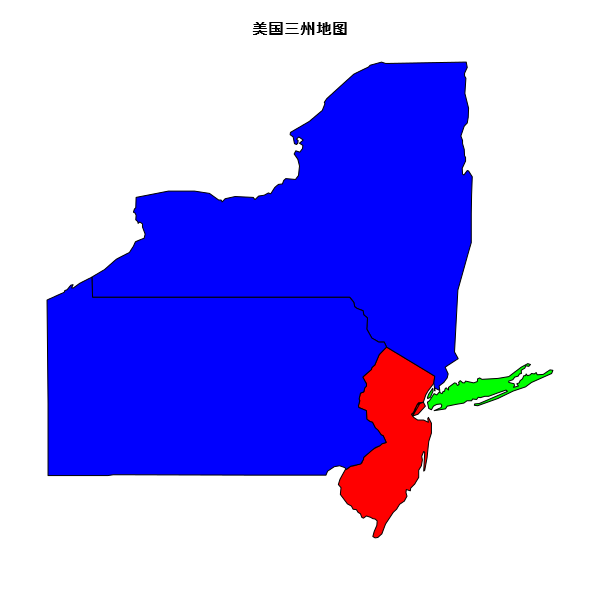## 3、 画中国地图

``````library(maps)
library(mapdata)
map("china", col = "red4", ylim = c(18, 54), panel.first = grid())
title(" 中国地图")
``````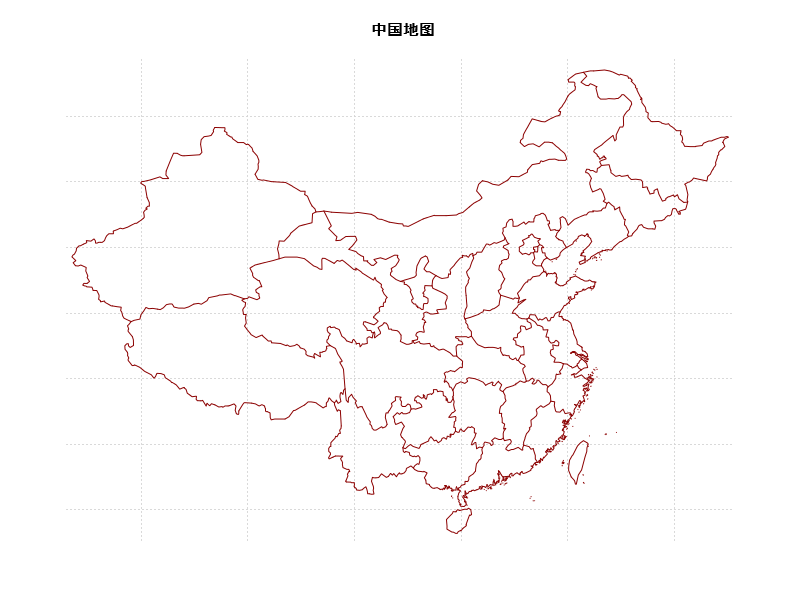## 1、geocode()

``````> geocode("Beijing")
lon      lat
1 116.4075 39.90403
``````

``````> #这意思就是大哥你多给点！！
> geocode("Renmin University of China", output = "more")
lon      lat              type     loctype
1 116.3184 39.96998 point_of_interest approximate
1 renmin university of china, 59号 zhongguancun street, haidian, beijing, china, 100086
north    south     east     west postal_code country
1 39.97853 39.96142 116.3345 116.3024      100086   china
1                        <NA>                     beijing  beijing
street streetNo          point_of_interest
1 zhongguancun street       NA renmin university of china
query
1 Renmin University of China
``````

## 2、mapdist()

``````> mapdist('China Agricultural University',
+     'Renmin University of China', 'walking')
from                         to    m    km
1 China Agricultural University Renmin University of China 6022 6.022
miles seconds  minutes    hours
1 3.742071    4523 75.38333 1.256389
``````

1 mile = 1609米。

``````library(ggmap)
library(mapproj)
map <- get_map(location = 'China', zoom = 4)
ggmap(map)
``````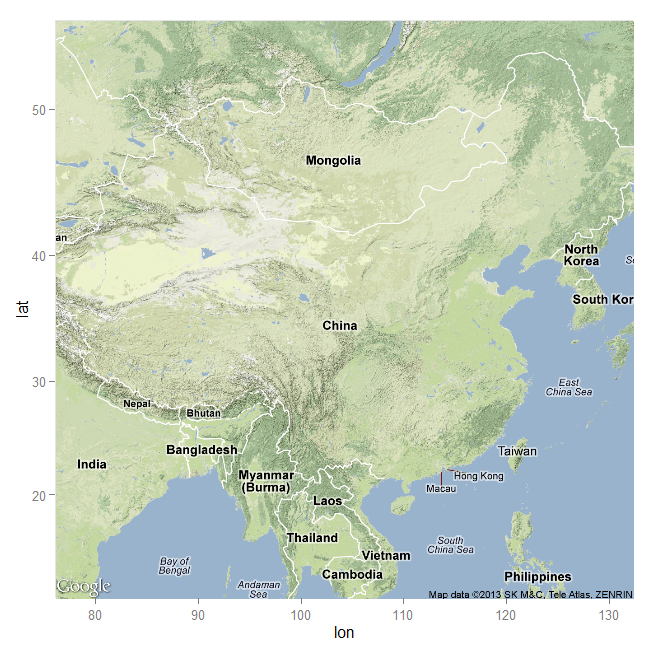``````#Google啊Google给我Beijing的公路地图数据吧
map <- get_map(location = 'Beijing', zoom = 10, maptype = 'roadmap')
ggmap(map)
``````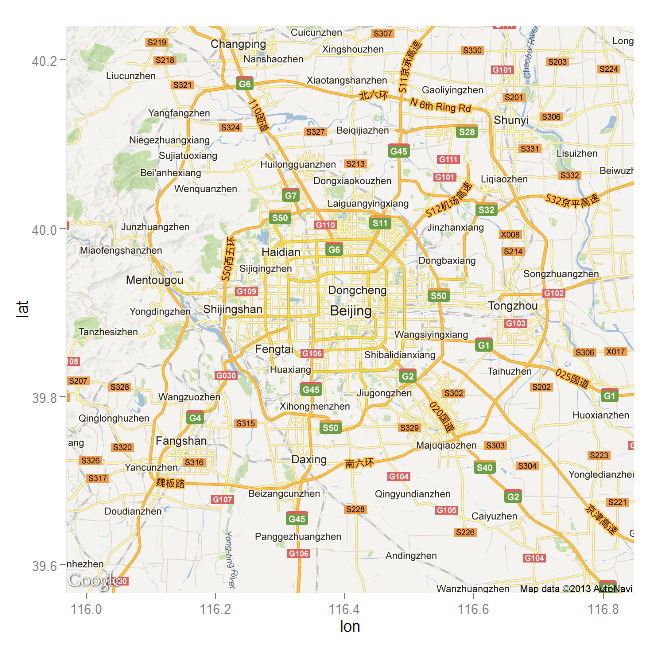``````## Google啊Google给我RUC的卫星地图数据吧
map <- get_map(location = 'Renmin University of China', zoom = 14,
maptype = 'satellite')
ggmap(map)
``````